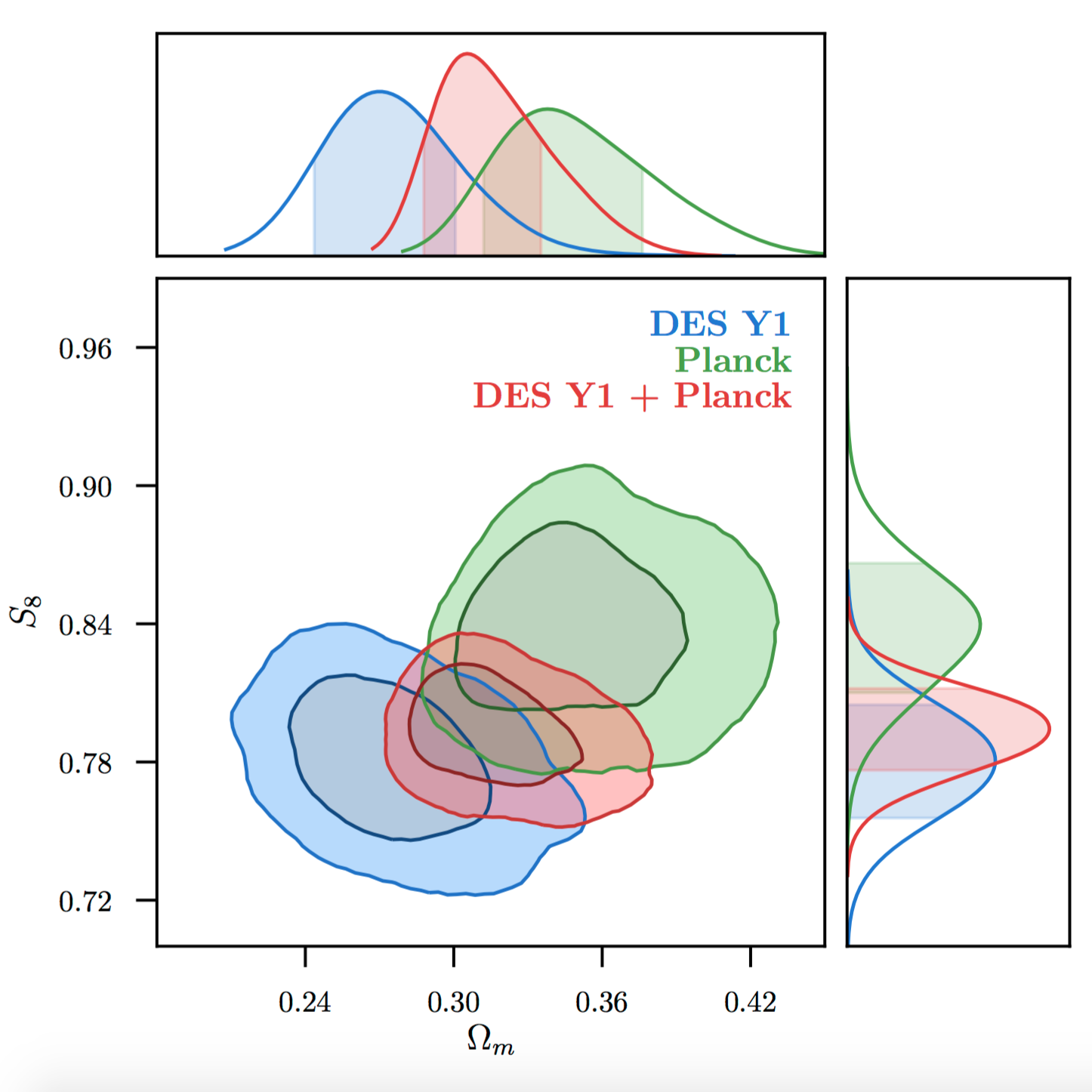# Dark Energy Survey Year 1 Results: Cosmological Constraints from Galaxy Clustering and Weak LensingWe present cosmological results from a combined analysis of galaxy clustering and weak gravitational lensing, using 1321 deg$^2$ of $griz$ imaging data from the first year of the Dark Energy Survey (DES Y1). We combine three two-point functions: (i) the cosmic shear correlation function of 26 million source galaxies in four redshift bins, (ii) the galaxy angular autocorrelation function of 650,000 luminous red galaxies in five redshift bins, and (iii) the galaxy-shear cross-correlation of luminous red galaxy positions and source galaxy shears. To demonstrate the robustness of these results, we use independent pairs of galaxy shape, photometric redshift estimation and validation, and likelihood analysis pipelines. To prevent confirmation bias, the bulk of the analysis was carried out while blind to the true results; we describe an extensive suite of systematics checks performed and passed during this blinded phase. The data are modeled in flat $\Lambda$CDM and $w$CDM cosmologies, marginalizing over 20 nuisance parameters, varying 6 (for $\Lambda$CDM) or 7 (for $w$CDM) cosmological parameters including the neutrino mass density and including the 457 $\times$ 457 element analytic covariance matrix. We find consistent cosmological results from these three two-point functions, and from their combination obtain $S_8 \equiv \sigma_8 (\Omega_m/0.3)^{0.5} = 0.783^{+0.021}_{-0.025}$ and $\Omega_m = 0.264^{+0.032}_{-0.019}$ for $\Lambda$CDM for $w$CDM, we find $S_8 = 0.794^{+0.029}_{-0.027}$, $\Omega_m = 0.279^{+0.043}_{-0.022}$, and $w=-0.80^{+0.20}_{-0.22}$ at 68% CL. The precision of these DES Y1 results rivals that from the Planck cosmic microwave background measurements, allowing a comparison of structure in the very early and late Universe on equal terms. Although the DES Y1 best-fit values for $S_8$ and $\Omega_m$ are lower than the central values from Planck ...